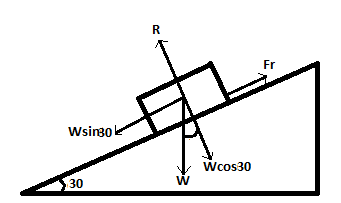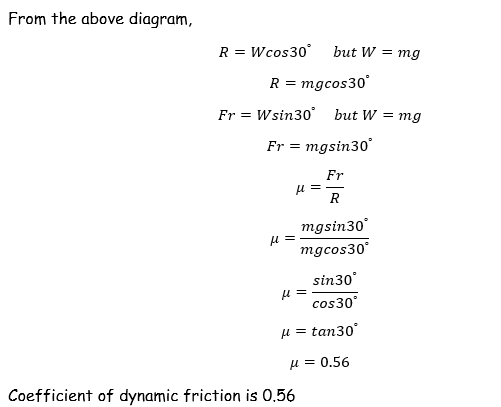error: Content is protected !!

# PHYSICS: FORM THREE: Topic 2 - FRICTION

TOPIC 2: FRICTION

The Concept of Friction
Explain the concept for friction
Friction is the force which opposes (resists) motion of the body. For example, a person will feel a certain resistance when pulling a block of wood resting on the table. The same kind of opposition can be felt when pushing a desk along a floor. That resistance (opposition) between two surfaces in contact is what we call friction.
Friction force is always in opposite direction to the applied force. This means, for a body to move, the applied force must exceed the friction force.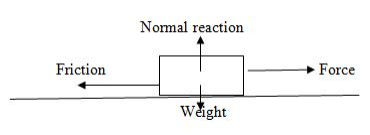Friction force between two surface in contact exists only when there is relative motion between the two contacting surface.
The friction between two surfaces exists because of the nature of the surface of the bodies in contact. The roughness of the surface is due to the fact that every surface consists of peaks and valleys.
Demonstration
The peak and valleys on the surfaces of bodies may be due to a random arrangement of coarse particles, for example the surface of a grinding stones.
Smooth surfaces exert almost no friction.
When one body is pushed against another, the peaks of one surface have to rise up over the peaks of the other surface. Hence, opposition to motion or friction occurs when the force is acting to restore the bodies to their original position.

Thefriction force has several advantages which includes the following:
1. It helps in walking process. In this process, friction force stop us from slipping over.
2. It helps cars to move on roads easily due to friction between car tyres and road.Therefore, makes the car stay on road. Most car tyres have deep dreads to increase friction between the tyres and the road surface.
3. It helps in car braking system. Brakes rely on friction between the brake drum or pads and the wheels. In a bicycle, there are brake pads which clamp onto the wheel to slow it down.
Frictional force has several dis-advantages which include the following:
1. Cause machinery to heat up and can cause wear and tear.
2. Cause machinery to be less efficient.
3. Cause machinery to produce noise.

Methods of Reducing Friction
Describe methods of reducing friction
The methods of reducing friction between surfaces include the following:
1. Polishing: Polishing the surface reduces the roughness and hence reduces friction.
2. Lubrication: It provides a layer of the smooth fluid or semi fluid between surfaces in contact so that they slide over each other smoothly.
3. Use of ball-bearings or rollers: Rollers help to convert sliding friction into a milder form-rolling friction. Rolling friction is lesser than sliding friction.
4. Streamlining: Fast cars, boats, planes etc., have a streamlined body. This is to allow air (or water in the case of boats) to easily flow by, without offering much resistance. Flying birds have streamlined bodies.
5. Use of correct combination of surfaces in contact: Use of alloys on moving and sliding parts reduces friction because alloys have a low coefficient of friction.
Rollers
• It is a simple cylinder on which a body to be pulled RESTS.
• It is used to eliminates sliding friction.
• Conveyor belts use metal rollers.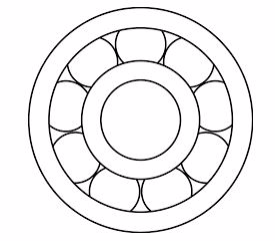Wheels
• These arerollers that are fixed to a moving body, held in place by cylinders or axles which are threaded in their centers. Example; a trailer towed by vehicle.
• Wheels are used to eliminate sliding friction.
• They are used to reduce friction (the friction between surfaces can be reduced by smoothing and polishing the surfaces in contact).
• In order to make surfaces slippery, a lubricant such as oil or graphite is used. Oil and Grease are commonly used in vehicles and machines to reduce friction between moving parts. In engines, differential air is also an effective lubricant in machine.
Types of Friction
Identify types of friction
There are three main types of friction in daily life which include the following:
1. Static friction: an opposing force between two solid objects at rest.In simple words, when there is no relative motion between two solid objects in contact with each other, we describe the frictional force between them asstatic.
2. Limiting friction: numerically equal to the minimum external force required to make a body just move over another.
3. Dynamic friction: numerically equal to the force of opposition when a body is moving over the rough surface.
Limiting Friction
Determine limiting friction
Limiting friction is the maximum frictional force existing between two bodies in contact. Is the maximum possible value of static friction. A force must be applied to overcome this limiting friction so as to enable the bodies move over one another. Therefore, limiting friction is equal to the minimum force required to make a body just move over one another. The limiting friction is independent of applied force but depends on nature of surface.
Example 1
A block of mass 20 kg is pulled along a horizontal surface. If the coefficient of friction is 0.4, what force is acting on the block?
Solution
Force = coefficient of friction ×mass × acceleration due to gravity
F = 0.420×10 = 80N.

Laws of Friction
State laws of friction
The five laws of friction
1. Friction force depends on the normal reaction (R). Friction force is direct proportional and perpendicular to the normal reaction.
2. Friction is independent of the area of contact.
3. The coefficient of static friction is slightly greater than the coefficient of kinetic friction.
4. Kinetic friction is independent of velocity of the body.
5. Friction depends on the nature of the surfaces in contact. Rough surfaces exert much friction compared to smooth surfaces.
The Coefficient of Friction
Determine the coefficient of friction
Coefficient of friction is the ratio of the frictional force that acts between two objects in contact to the normal reaction, R.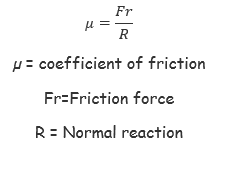Types of Coefficient of friction
Coefficient of friction can be static or dynamic.
Coefficient of static friction is the ratio of the static friction to the normal reaction. This is obtained when the body is trying to start a motion but not yet moving.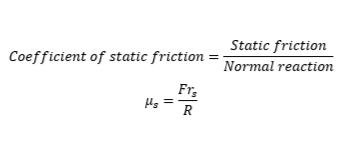Coefficient of dynamic friction is the ratio of the dynamic friction to the normal reaction that acts on the body. This is obtained when the body is in motion. Coefficient of dynamic friction is slightly smaller than the coefficient of static friction.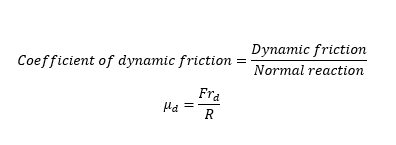Laws of Friction in Solving Problems
Apply laws of friction in solving problems
Consider a rectangular block sliding down the inclined plane of angle Q0. The block is of weight Wand is sliding freely using its weight component WsinQ. This weight component is opposed by a friction force Fr. The normal reaction is perpendicular to the inclined plane and is opposite to the weight component WcosQ of the block.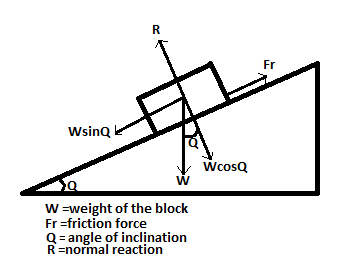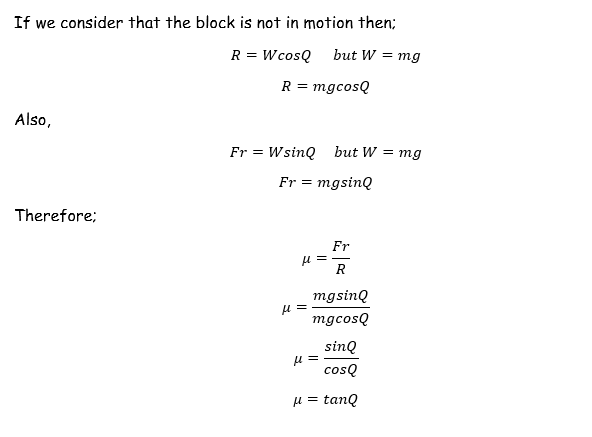Example 2
Find the static friction between a block of wood of mass 10kg and the table on which it rests. A minimum force of 50N is required to make the block just move on the table top.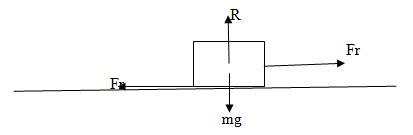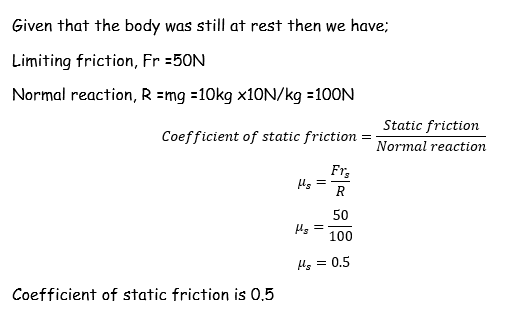Example 3
A mass is placed on an Inclined plane such that it moves at a constant speed when tapped tightly. If the angle the plane makes with the horizontal is 30º. Find the coefficient of dynamic friction.